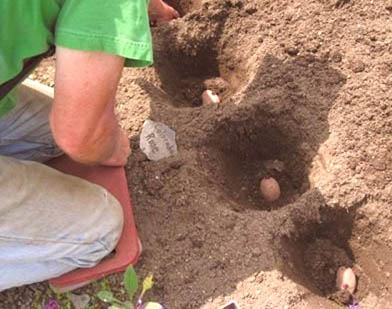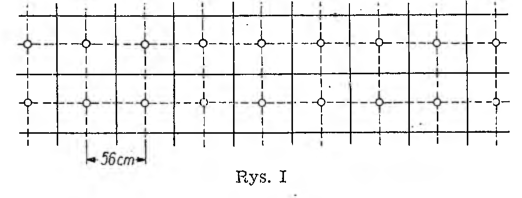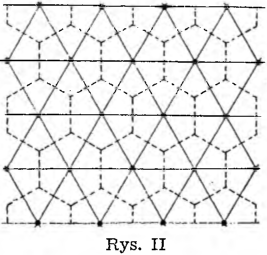# About the most favorable method of planting potatoesSo that the potatoes yield the maximum yield, they should be planted at a certain constant distance prescribed by science based on many years of experience. The point is, however, what will be the most advantageous placement in the field. And on this matter, not only agronomy has something to say, but also mathematics.

As you know, there are three such polygons, into which the plane can be broken without gaps or gaps, namely: equilateral triangle, square and regular hexagon. Thus, only these three types of relative potato distribution can be considered. That when using a hexagon (in view of the necessary constant minimum distance between the potatoes) the soil will not be sufficiently exploited, it is almost obvious. Doubts can only arise when choosing a triangle or a square. In each of these polygons, we will put one plant in the center, and we will select the dimensions of the rectangles in this way, that the distance between the plants closest to each other is of the prescribed size, e.g.. d — 56 cm. (If someone wanted to perform calculations for a different value of d, he won't have much trouble).When planting potatoes in a "square", as shown in figure I, we have to arrange the rows at intervals after 56 cm and plant every row in each row 56 cm. There will be for every plant 56 • 56 = 3136cm² of the ground, and on one ara (10X10 m) we will plant 1 000 000 : 3136 = 319 plants.

Let us also consider the method of planting potatoes in the vertices of equilateral triangles (Lynx. II).With this method of planting, each plant will have a regular hexagon, in which the distance between the center and the sides will be 28 cm. The whole regular hexagon can be broken down into 6 equilateral triangles.

In each of these triangles, the height is 28 cm, and the side - as it is easy to calculate - approx 32 cm. The area of ​​such a small triangle is ½ • 32 • 28 = 448 cm², and the area of ​​the whole regular hexagon is 6 • 448 = 2688 cm². You can drop off on one aara 1 000 000 : 2688 = 372 plants, which is more than when planting in a square.